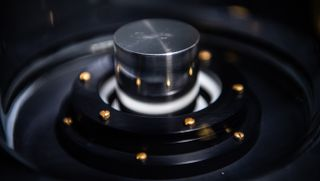# There's a Brand-New Kilogram, And It's Based on Quantum PhysicsThe kilogram isn't a thing anymore. Instead, it's an abstract idea about light and energy.

As of today (May 20), physicists have replaced the old kilogram — a 130-year-old, platinum-iridium cylinder weighing 2.2 pounds (1 kilogram) sitting in a room in France —— with an abstract, unchanging measurement based on quadrillions of light particles and Planck's constant (a fundamental feature of our universe).

In one sense, this is a grand (and surprisingly difficult) achievement. The kilogram is fixed forever now. It can't change over time as the cylinder loses an atom here or an atom there. That means humans could communicate this unit of mass, in terms of raw science, to space aliens. The kilogram is now a simple truth, an idea that can be carried anywhere in the universe without bothering to bring a cylinder with you.

And still...so what? Practically speaking, the new kilogram weighs, to within a few parts per billion, exactly as much as the old kilogram did. If you weighed 93 kilograms (204 pounds) yesterday, you'll weigh 93 kilograms today and tomorrow. Only in a few narrow scientific applications will the new definition make any difference. [7 Strange Facts About Quarks]

What's really fascinating here isn't that, practically speaking, the way most of us use the kilogram will change. It's how damn difficult it turned out to be to rigorously define a unit of mass at all.

Other fundamental forces have long since been understood in terms of fundamental reality. A second of time? Once, according to the National Institute of Standards and Technology (NIST), it was defined in terms of the swings of a pendulum clock. But now scientists understand a second as the time it takes an atom of cesium 133 to go through 9,192,631,770 cycles of releasing microwave radiation. A meter? That's the distance light travels in 1/299,792,458th of a second.

But mass isn't like that. We usually measure kilograms in terms of weight — how much does this thing push down on a scale? But that's a measurement that depends on where you perform the actual weighing. That cylinder in France would weigh much less if you brought it to the moon, and even a tiny bit more or tiny bit less if you brought it to other parts of the Earth.

As NIST explains, the new kilogram is based on the fundamental relationship between mass and energy — the relationship partly spelled out in Einstein's E=mc^2, which means energy is equal to mass times the speed of light squared. Mass can be converted to energy and vice versa. And, compared with mass, energy is easier to measure and define in discrete terms.

That's thanks to another equation, even older than E=mc^2. The physicist Max Planck showed in 1900 that E=hv, according to NIST. He showed that, on a small enough scale, energy can go up and down, and only in steps. E=hv means that energy is equal to "v" — the frequency of some particle, like a photon — multiplied by "h" — the number 6.62607015×10^minus34 also known as Planck's constant.

"v" in E=hv must always be an integer, like 1, 2, 3 or 6,492. No fractions or decimals allowed. So, energy is by its nature discrete, going up and down in steps of "h" (6.62607015×10^minus34).

The new kilogram brings E=mc^2 and E=hv together. That enables scientists to define mass in terms of Planck's constant, an unchanging feature of the universe. An international coalition of science labs came together to make the most precise measurements of Planck's constant yet, certain to within just several parts per billion. The new kilogram's mass corresponds to the energy of 1.4755214 times 10^40 photons that are oscillating at the same frequencies as the cesium 133 atoms used in atomic clocks.

It's not the easiest thing to stick on a scale. But, as an idea, it's a lot more portable than a cylinder of platinum-iridium alloy.

Originally published on Live Science.